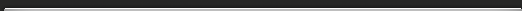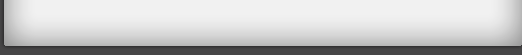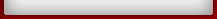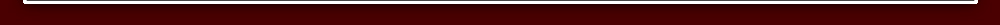Tutorial 6

## Tutorial 6

Multiplying a number by 11
To multiply any 2-figure number by 11 we just put the total of the two figures between the 2 figures.
26 x 11 = 286
Notice that the outer figures in 286 are the 26 being multiplied.
And the middle figure is just 2 and 6 added up.
So 72 x 11 = 792
Exercise 1 Tutorial 6

Multiply by 11:

1) 43 =
2) 81 =
3) 15 =
4) 44 =
5) 11 =

77 x 11 = 847 This involves a carry figure because 7 + 7 = 14
we get 77 x 11 = 7147 = 847.

Exercise 2 Tutorial 6
Multiply by 11:

1) 11 x 88 =
2) 11 x 84 =
3) 11 x 48 =
4) 11 x 73 =
5) 11 x 56 =

234 x 11 = 2574
We put the 2 and the 4 at the ends.
We add the first pair 2 + 3 = 5.
and we add the last pair: 3 + 4 = 7.

Exercise 3 Tutorial 6
Multiply by 11:

1) 151 =
2) 527 =
3) 333 =
4) 714 =
5) 909 =